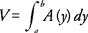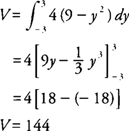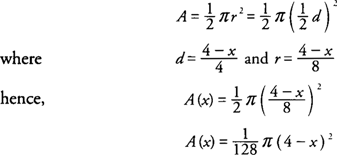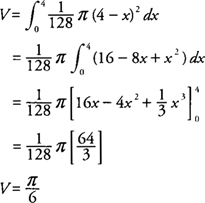## Volumes of Solids with Known Cross Sections

You can use the definite integral to find the volume of a solid with specific cross sections on an interval, provided you know a formula for the region determined by each cross section. If the cross sections generated are perpendicular to the x‐axis, then their areas will be functions of x, denoted by A(x). The volume ( V) of the solid on the interval [ a, b] isIf the cross sections are perpendicular to the y‐axis, then their areas will be functions of y, denoted by A(y). In this case, the volume ( V) of the solid on [ a, b] isExample 1: Find the volume of the solid whose base is the region inside the circle x 2 + y 2 = 9 if cross sections taken perpendicular to the y‐axis are squares.

Because the cross sections are squares perpendicular to the y‐axis, the area of each cross section should be expressed as a function of y. The length of the side of the square is determined by two points on the circle x 2 + y 2 = 9 (Figure 1).Figure 1 Diagram for Example 1.

The area ( A) of an arbitrary square cross section is A = s 2, whereThe volume ( V) of the solid isExample 2: Find the volume of the solid whose base is the region bounded by the lines x + 4 y = 4, x = 0, and y = 0, if the cross sections taken perpendicular to the x‐axis are semicircles.

Because the cross sections are semicircles perpendicular to the x‐axis, the area of each cross section should be expressed as a function of x. The diameter of the semicircle is determined by a point on the line x + 4 y = 4 and a point on the x‐axis (Figure 2).Figure 2 Diagram for Example 2.

The area ( A) of an arbitrary semicircle cross section isThe volume ( V) of the solid is